Tamil Nadu Board of Secondary EducationSSLC (English Medium) Class 7th

# Tamil Nadu Board Samacheer Kalvi solutions for Class 7th Mathematics Term 1 Answers Guide chapter 5 - Geometry [Latest edition]

#### Chapters## Chapter 5: Geometry

Exercise 5.1Exercise 5.2Exercise 5.3Exercise 5.4Exercise 5.5Exercise 5.6
Exercise 5.1 [Pages 92 - 93]

### Tamil Nadu Board Samacheer Kalvi solutions for Class 7th Mathematics Term 1 Answers Guide Chapter 5 Geometry Exercise 5.1 [Pages 92 - 93]

Exercise 5.1 | Q 1 | Page 92

Name the pairs of adjacent angles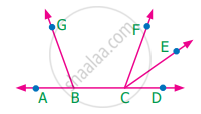Exercise 5.1 | Q 2 | Page 92

Find the angle ∠JIL from the given figure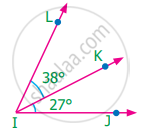Exercise 5.1 | Q 3 | Page 92

Find the angles ∠GEH from the given figure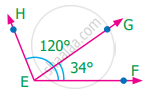Exercise 5.1 | Q 4. (i) | Page 92

Given that AB is a straight line. Calculate the value of x° in the following cases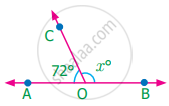Exercise 5.1 | Q 4. (ii) | Page 92

Given that AB is a straight line. Calculate the value of x° in the following cases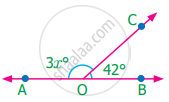Exercise 5.1 | Q 4. (iii) | Page 92

Given that AB is a straight line. Calculate the value of x° in the following cases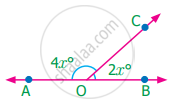Exercise 5.1 | Q 5 | Page 92

One angle of a linear pair is a right angle. What can you say about the other angle?

Exercise 5.1 | Q 6 | Page 92

If the three angles at a point are in the ratio 1 : 4 : 7, find the value of each angle?

Exercise 5.1 | Q 7 | Page 92

There are six angles at a point. One of them is 45° and the other five angles are all equal. What is the measure of all the five angles?

Exercise 5.1 | Q 8. (i) | Page 92

In the given figure, identify any two pairs of adjacent angles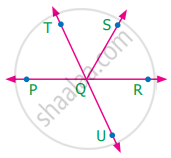Exercise 5.1 | Q 8. (ii) | Page 92

In the given figure, identify two pairs of vertically opposite anglesExercise 5.1 | Q 9 | Page 92

The angles at a point are x°, 2x°, 3x°, 4x° and 5x°. Find the value of the largest angle?

Exercise 5.1 | Q 10 | Page 92

From the given figure, find the missing angle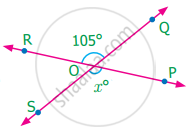Exercise 5.1 | Q 11 | Page 92

Find the angles x° and y° in the figure shown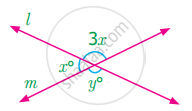Exercise 5.1 | Q 12 | Page 92

Using the figure, answer the following questions

(i) What is the measure of angle x°?
(ii) What is the measure of angle y°?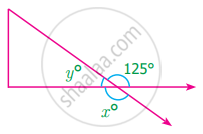#### Objective type questions

Exercise 5.1 | Q 13 | Page 93

• no common interior, no common arm, no common vertex.

• one common vertex, one common arm, common interior

• one common arm, one common vertex, no common interior.

• one common arm, no common vertex, no common interior.

Exercise 5.1 | Q 14 | Page 93

In the given figure the angles ∠1 and ∠2 are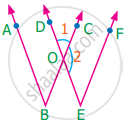• Opposite angles

• Linear pair

• Supplementary angles

Exercise 5.1 | Q 15 | Page 93

Vertically opposite angles are

• not equal in measure

• complementary

• supplementary

• equal in measure

Exercise 5.1 | Q 16 | Page 93

The sum of all angles at a point is

• 360°

• 180°

• 90°

Exercise 5.1 | Q 17 | Page 93

The measure of ∠BOC is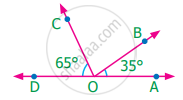• 90°

• 180°

•  80°

• 100°

Exercise 5.2 [Pages 100 - 102]

### Tamil Nadu Board Samacheer Kalvi solutions for Class 7th Mathematics Term 1 Answers Guide Chapter 5 Geometry Exercise 5.2 [Pages 100 - 102]

Exercise 5.2 | Q 1. (i) | Page 100

From the figure name the marked pair of angles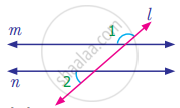Exercise 5.2 | Q 1. (ii) | Page 100

From the figure name the marked pair of angles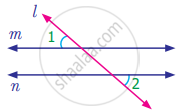Exercise 5.2 | Q 1. (iii) | Page 100

From the figure name the marked pair of angles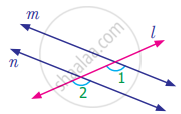Exercise 5.2 | Q 1. (iv) | Page 100

From the figure name the marked pair of angles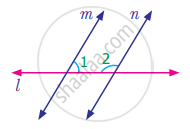Exercise 5.2 | Q 1. (v) | Page 100

From the figure name the marked pair of angles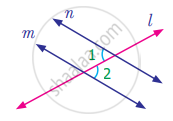Exercise 5.2 | Q 1. (vi) | Page 100

From the figure name the marked pair of angles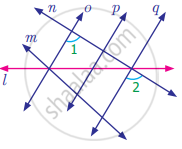Exercise 5.2 | Q 2. (i) | Page 100

Find the measure of angle x in the following figure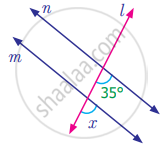Exercise 5.2 | Q 2. (ii) | Page 100

Find the measure of angle x in the following figure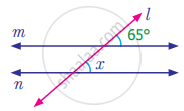Exercise 5.2 | Q 2. (iii) | Page 100

Find the measure of angle x in the following figure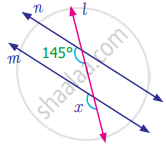Exercise 5.2 | Q 2. (iv) | Page 100

Find the measure of angle x in the following figure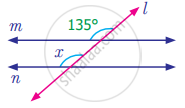Exercise 5.2 | Q 2. (v) | Page 100

Find the measure of angle x in the following figure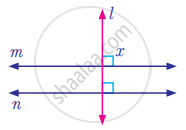Exercise 5.2 | Q 3. (i) | Page 100

Find the measure of angle y in the following figure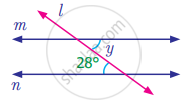Exercise 5.2 | Q 3. (ii) | Page 100

Find the measure of angle y in the following figure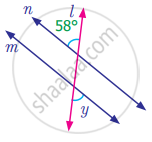Exercise 5.2 | Q 3. (iii) | Page 100

Find the measure of angle y in the following figure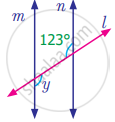Exercise 5.2 | Q 3. (iv) | Page 100

Find the measure of angle y in the following figure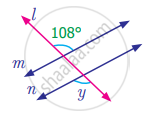Exercise 5.2 | Q 4. (i) | Page 101

Find the measure of angle z in the following figure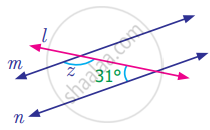Exercise 5.2 | Q 4. (ii) | Page 101

Find the measure of angle z in the following figure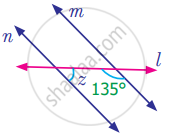Exercise 5.2 | Q 4. (iii) | Page 101

Find the measure of angle z in the following figure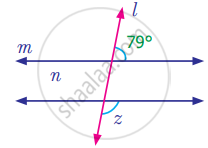Exercise 5.2 | Q 4. (iv) | Page 101

Find the measure of angle z in the following figure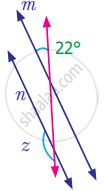Exercise 5.2 | Q 5. (i) | Page 101

Find the value of angle a in the following figure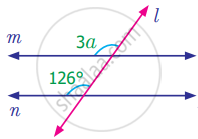Exercise 5.2 | Q 5. (ii) | Page 101

Find the value of angle a in the following figure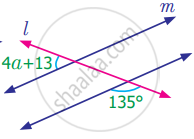Exercise 5.2 | Q 5. (iii) | Page 101

Find the value of angle a in the following figure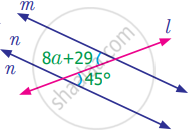Exercise 5.2 | Q 5. (iv) | Page 101

Find the value of angle a in the following figure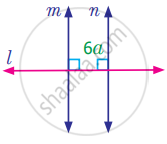Exercise 5.2 | Q 6. (i) | Page 101

Find the value of angle x in the following figure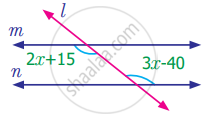Exercise 5.2 | Q 6. (ii) | Page 101

Find the value of angle x in the following figure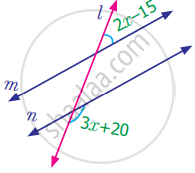Exercise 5.2 | Q 7. (i) | Page 101

Anbu has marked the angles as shown in the following figure. Check whether it is correct. Give reason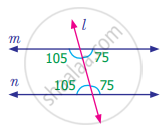Exercise 5.2 | Q 7. (ii) | Page 101

Anbu has marked the angles as shown in the following figure. Check whether it is correct. Give reason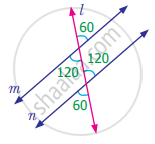Exercise 5.2 | Q 8 | Page 101

Mention two real life situations where we use parallel lines

Exercise 5.2 | Q 9 | Page 101

Two parallel lines are intersected by a transversal. What is the minimum number of angles you need to know to find the remaining angles. Give reason

#### Objective type questions

Exercise 5.2 | Q 10 | Page 101

A line which intersects two or more lines in different points is known as

• parallel lines

• transversal

• non-parallel lines

• Intersecting lines

Exercise 5.2 | Q 11 | Page 101

In the given figure angles a and b are

• alternate exterior angles

• corresponding angles

• alternate interior angles

• vertically opposite angles

Exercise 5.2 | Q 12 | Page 102

Which of the following statement is ALWAYS TRUE when parallel lines are cut by a transversal

• corresponding angles are supplementary.

• alternate interior angles are supplementary.

• alternate exterior angles are supplementary.

• interior angles on the same side of the transversal are supplementary.

Exercise 5.2 | Q 13 | Page 102

In the diagram what is the value of angle x?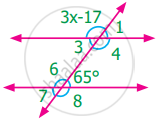• 43°

• 44°

• 132°

• 134°

Exercise 5.3 [Page 103]

### Tamil Nadu Board Samacheer Kalvi solutions for Class 7th Mathematics Term 1 Answers Guide Chapter 5 Geometry Exercise 5.3 [Page 103]

Exercise 5.3 | Q 1. (a) | Page 103

Draw a line segment of given length and construct a perpendicular bisector to line segment using scale and compass

8 cm

Exercise 5.3 | Q 1. (b) | Page 103

Draw a line segment of given length and construct a perpendicular bisector to line segment using scale and compass

7 cm

Exercise 5.3 | Q 1. (c) | Page 103

Draw a line segment of given length and construct a perpendicular bisector to line segment using scale and compass

5.6 cm

Exercise 5.3 | Q 1. (d) | Page 103

Draw a line segment of given length and construct a perpendicular bisector to line segment using scale and compass

10.4 cm

Exercise 5.3 | Q 1. (e) | Page 103

Draw a line segment of given length and construct a perpendicular bisector to line segment using scale and compass

58 mm

Exercise 5.4 [Page 104]

### Tamil Nadu Board Samacheer Kalvi solutions for Class 7th Mathematics Term 1 Answers Guide Chapter 5 Geometry Exercise 5.4 [Page 104]

Exercise 5.4 | Q 1. (a) | Page 104

Construct the following angles using protractor and draw a bisector to the angle using ruler and compass

60°

Exercise 5.4 | Q 1. (b) | Page 104

Construct the following angles using protractor and draw a bisector to the angle using ruler and compass

100°

Exercise 5.4 | Q 1. (c) | Page 104

Construct the following angles using protractor and draw a bisector to the angle using ruler and compass

90°

Exercise 5.4 | Q 1. (d) | Page 104

Construct the following angles using protractor and draw a bisector to the angle using ruler and compass

48°

Exercise 5.4 | Q 1. (e) | Page 104

Construct the following angles using protractor and draw a bisector to the angle using ruler and compass

110°

Exercise 5.5 [Page 105]

### Tamil Nadu Board Samacheer Kalvi solutions for Class 7th Mathematics Term 1 Answers Guide Chapter 5 Geometry Exercise 5.5 [Page 105]

Exercise 5.5 | Q 1. (i) | Page 105

Construct the following angle using ruler and compass only

60°

Exercise 5.5 | Q 1. (ii) | Page 105

Construct the following angle using ruler and compass only

120°

Exercise 5.5 | Q 1. (iii) | Page 105

Construct the following angle using ruler and compass only

30°

Exercise 5.5 | Q 1. (iv) | Page 105

Construct the following angle using ruler and compass only

90°

Exercise 5.5 | Q 1. (v) | Page 105

Construct the following angle using ruler and compass only

45°

Exercise 5.5 | Q 1. (vi) | Page 105

Construct the following angle using ruler and compass only

150°

Exercise 5.5 | Q 1. (vii) | Page 105

Construct the following angle using ruler and compass only

135°

Exercise 5.6 [Pages 105 - 107]

### Tamil Nadu Board Samacheer Kalvi solutions for Class 7th Mathematics Term 1 Answers Guide Chapter 5 Geometry Exercise 5.6 [Pages 105 - 107]

#### Miscellaneous Practice problems

Exercise 5.6 | Q 1 | Page 105

Find the value of x if ∠AOB is a right angle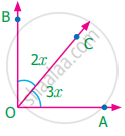Exercise 5.6 | Q 2 | Page 105

In the given figure, find the value of xExercise 5.6 | Q 3 | Page 105

Find the value of x, y and z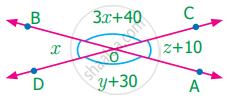Exercise 5.6 | Q 4 | Page 105

Two angles are in the ratio 11 : 25. If they are linear pair, find the angles

Exercise 5.6 | Q 5. (i) | Page 105

Using the figure, answer the following question and justify your answer

Is ∠1 adjacent to ∠2?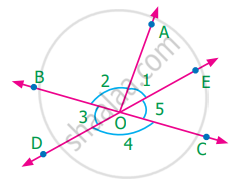Exercise 5.6 | Q 5. (ii) | Page 105

Using the figure, answer the following question and justify your answer

Is ∠AOB adjacent to ∠BOE?Exercise 5.6 | Q 5. (iii) | Page 105

Using the figure, answer the following question and justify your answer

Does ∠BOC and ∠BOD form a linear pair?Exercise 5.6 | Q 5. (iv) | Page 105

Using the figure, answer the following question and justify your answer

Are the angles ∠COD and ∠BOD supplementary?Exercise 5.6 | Q 5. (v) | Page 105

Using the figure, answer the following question and justify your answer

Is ∠3 vertically opposite to ∠1?Exercise 5.6 | Q 6 | Page 106

In the figure POQ, ROS and TOU are straight lines. Find the x°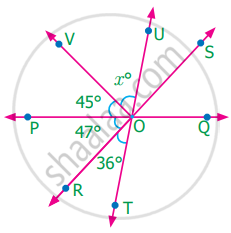Exercise 5.6 | Q 7 | Page 106

In the figure AB is parallel to DC. Find the value of ∠1 and ∠2. Justify your answer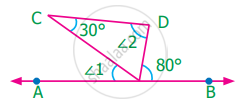Exercise 5.6 | Q 8 | Page 106

In the figure AB is parallel to CD. Find x, y and z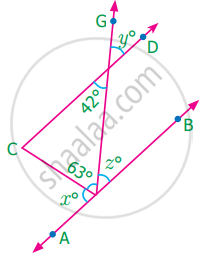Exercise 5.6 | Q 9 | Page 106

Draw two parallel lines and a transversal. Mark two alternate interior angles G and H. If they are supplementary, what is the measure of each angle?

Exercise 5.6 | Q 10 | Page 106

A plumber must install pipe 2 parallel to pipe 1. He knows that ∠1 is 53. What is the measure of ∠2?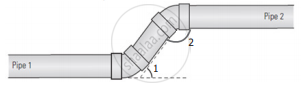#### Challenge Problems

Exercise 5.6 | Q 11 | Page 106

Find the value of y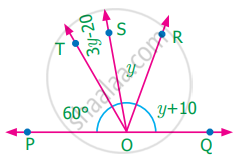Exercise 5.6 | Q 12 | Page 106

Find the value of z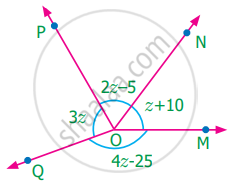Exercise 5.6 | Q 13 | Page 106

Find the value of x and y if RS is parallel to PQ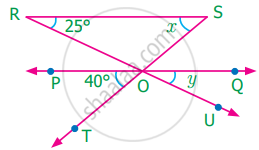Exercise 5.6 | Q 14 | Page 106

Two parallel lines are cut by a transversal. For each pair of interior angles on the same side of the transversal, if one angle exceeds the twice of the other angle by 48°. Find the angles

Exercise 5.6 | Q 15 | Page 106

In the figure, the lines GH and IJ are parallel. If ∠1 = 108° and ∠2 = 123°, find the value of x, y and z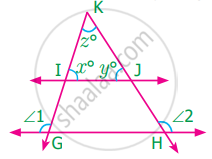Exercise 5.6 | Q 16 | Page 106

In the parking lot shown, the lines that mark the width space are parallel. If ∠1 =(x + 39)°, ∠2 = (2x – 3y)° find x and y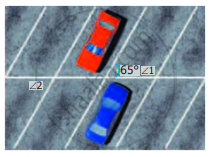Exercise 5.6 | Q 17 | Page 107

Draw two parallel lines and a transversal. Mark two corresponding angles A and B. If ∠A = 4x, and ∠B = 3x + 7, find the value of x. Explain

Exercise 5.6 | Q 18 | Page 107

In the figure AB in parallel to CD. Find x°, y° and z°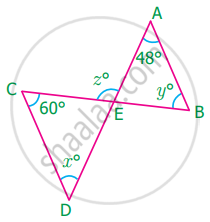Exercise 5.6 | Q 19 | Page 107

Two parallel lines are cut by transversal. If one angle of a pair of corresponding angles can be represented by 42° less than three times the other. Find the corresponding angles

Exercise 5.6 | Q 20 | Page 107

In the given figure, ∠8 = 107°, what is the sum of the ∠2 and ∠4?

## Chapter 5: Geometry

Exercise 5.1Exercise 5.2Exercise 5.3Exercise 5.4Exercise 5.5Exercise 5.6## Tamil Nadu Board Samacheer Kalvi solutions for Class 7th Mathematics Term 1 Answers Guide chapter 5 - Geometry

Tamil Nadu Board Samacheer Kalvi solutions for Class 7th Mathematics Term 1 Answers Guide chapter 5 (Geometry) include all questions with solution and detail explanation. This will clear students doubts about any question and improve application skills while preparing for board exams. The detailed, step-by-step solutions will help you understand the concepts better and clear your confusions, if any. Shaalaa.com has the Tamil Nadu Board of Secondary Education Class 7th Mathematics Term 1 Answers Guide solutions in a manner that help students grasp basic concepts better and faster.

Further, we at Shaalaa.com provide such solutions so that students can prepare for written exams. Tamil Nadu Board Samacheer Kalvi textbook solutions can be a core help for self-study and acts as a perfect self-help guidance for students.

Concepts covered in Class 7th Mathematics Term 1 Answers Guide chapter 5 Geometry are Concept of Line, Concept of Line Segment, Concept of Ray, Parallel Lines, Concept of Intersecting Lines, Concept of Points, Concept of Angle - Arms, Vertex, Interior and Exterior Region, Types of Angles- Acute, Obtuse, Right, Straight, Reflex, Complete and Zero Angle., Complementary Angles, Supplementary Angles, Adjacent Angles, Concept of Linear Pair, Concept of Vertically Opposite Angles, Pairs of Lines - Transversal, Pairs of Lines - Angles Made by a Transversal, Pairs of Lines - Transversal of Parallel Lines, Drawing the Perpendicular Bisector of a Line Segment, Constructing a Bisector of an Angle, Angles of Special Measures - 30°, 45°, 60°, 90°, and 120°.

Using Tamil Nadu Board Samacheer Kalvi Class 7th solutions Geometry exercise by students are an easy way to prepare for the exams, as they involve solutions arranged chapter-wise also page wise. The questions involved in Tamil Nadu Board Samacheer Kalvi Solutions are important questions that can be asked in the final exam. Maximum students of Tamil Nadu Board of Secondary Education Class 7th prefer Tamil Nadu Board Samacheer Kalvi Textbook Solutions to score more in exam.

Get the free view of chapter 5 Geometry Class 7th extra questions for Class 7th Mathematics Term 1 Answers Guide and can use Shaalaa.com to keep it handy for your exam preparation